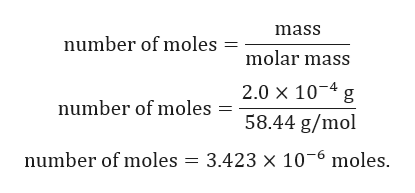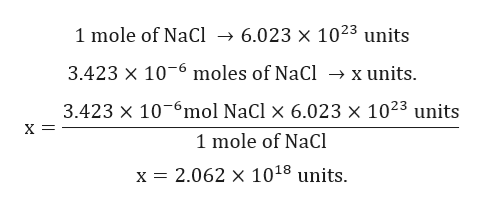A salt crystal has a mass of 0.20 mg, How many NaCl formula units does it contain?

Question

A salt crystal has a mass of 0.20 mg, How many NaCl formula units does it contain?

Step 1

Given:

Mass of NaCl = 0.20 mg = 2.0x10-4 g.

Molar mass of NaCl = 58.44 g/mol.

Step 2

Calculation for number of moles of NaCl:help_outlineImage Transcriptionclosemass number of moles molar mass 2.0 x 10 4 g number of moles 58.44 g/mol number of moles = 3.423 x 10-6 moles fullscreen
Step 3

Calculation for N...help_outlineImage Transcriptionclose6.023 x 1023 units 1 mole of NaCl 3.423 x 10 x units moles of NaCl 3.423 x 106mol NaCl x 6.023 x 1023 units 1 mole of NaCl x = 2.062 x 1018 units fullscreen

Want to see the full answer?

See Solution

Want to see this answer and more?

Our solutions are written by experts, many with advanced degrees, and available 24/7

See Solution
Tagged in

Chemistry